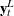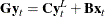The SIMLIN Procedure

Defining the Structural Form

An EST= input data set supplies the coefficients of the equation system. The data set containing the coefficients is normally a "TYPE=EST" data set created by the OUTEST= option of PROC SYSLIN or another regression procedure. The data set contains the special variables _TYPE_, _DEPVAR_, and INTERCEPT. You can also supply the structural coefficients of the system to PROC SIMLIN in a data set produced by a SAS DATA step as long as the data set is of the form TYPE=EST. Refer to SAS/STAT software documentation for a discussion of the special TYPE=EST type of SAS data set.

Suppose that there is a g1 vector of endogenous variables y, an l1 vector of lagged endogenous variables, and a k1 vector of exogenous variables x, including the intercept. Then, there are g structural equations in the simultaneous system that can be writtenwhere G is the matrix of coefficients of current period endogenous variables, C is the matrix of coefficients of lagged endogenous variables, and B is the matrix of coefficients of exogenous variables. G is assumed to be nonsingular.